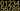## 持续最久的数字乘积

Wed, 29th January 2020Edit on Github数字

# 问题描述

2009年2月medie2005提出问题:

# 具体进展

## 前10个结果

P(11) = 1
P(29) = 2
P(47) = 3
P(277) = 4
P(769) = 5
P(8867) = 6
P(186889) = 7
P(2678789) = 8
P(26899889) = 9
P(3777888899) = 10 #云梦指出这个数不是素数

## 算法分析

medie2005建议穷举只含素因子2,3,5,7的数,对某个$n=2^a3^b5^c7^d$,计算n的十进制表示的数字的乘积s,然后n到s就有一条边.

## 11阶结果

mathe估算出$10^{60}$以内这样数的数目约等于$\frac1{4!}\frac{\log^4(10^{60})}{\log(2)\log(3)\log(5)\log(7)}\approx 6364870.77$

## 是否存在12阶结果

medie2005和mathe继续寻找12阶的素数，但是他们穷举到$10^{200}$以内范围还是没有找到结果

$(\frac45)^{5n}\approx \exp(-n)$.

$\int_{n0}^{\infty}\exp(-n)d1.37n^3=4.11\int_{n0}^{\infty}x^2\exp(-x)dx=(2+2n0+n0^2)\exp(-n0)$.

n0=10时大概为0.0055. n0=20时大概为$9.1\times 10^{-7}$.
n0=100时大概为$3.8\times 10^{-40}$.

gxqcn统计了（应该是不超过22位？）形如$2^p 3^q 7^r$$3^q 5^s 7^r$系列整数的持续数步数:

Github